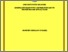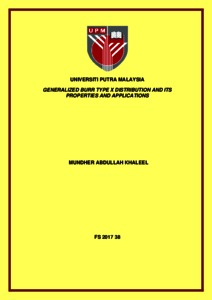# Generalized Burr type X distribution and its properties and applications

## Citation

Khaleel, Mundher Abdullah (2017) Generalized Burr type X distribution and its properties and applications. Doctoral thesis, Universiti Putra Malaysia.

## Abstract

We develop three new models from the baseline Burr type X with two parameters distribution (BX) using the beta-G, gamma-G and Weibull-G families of distributions. Burr type X distribution is chosen because the probability density function (pdf) and the cumulative distribution function (cdf) are of closed form. As a consequence of this, it can be used very conveniently even for censored data. Unlike Weibull, Generalized Exponential and gamma distributions, BX has a nonmonotone hazard function, which is suitable for the applications for right skewed data. Thus, the new models are attractive in terms of flexibility in dealing with right skewed, left skewed and approximately symmetric data. These three new distributions are known as beta Burr type X distribution (BBX),gamma Burr type X distribution (GBX) and Weibull Burr type X distribution (WBX).For each of the new distributions, the probability density function and failure rate function are found to be more flexible in accommodating different shapes. The new distributions have numerous sub-models as special cases and the expansions of pdf and cdf for each are obtained. We derived several mathematical properties of BBX, GBX andWBX distributions which include the quantile function, rth moment, moment generating function, mean deviation, R ́enyi entropy and order statistics. The maximum likelihood method is employed for the estimation of the parameters of BBX, GBX and WBX distributions. Simulation studies are carried out for varying sample sizes and parameter values to assess the performance of BBX, GBX and WBX distributions. Real data sets are used to illustrate the flexibility of BBX, GBX and WBX distributions. From the results, each distribution has provided a better fit than its sub-models and nonnested models based on the criteria such as the Akaike information criterion(AIC), corrected Akaike information criterion(CAIC), Bayesian information criterion(BIC), Hannan-Quinn information criterion(HQIC)and Kolmogorov-Smirnov statistic(K−S). From the real data analyses, WBX is found to fit left skewed and approximately symmetric data best while GBX fits the right skewed data bestPreview
Text
FS 2017 38 IR.pdfView Item